# 最大流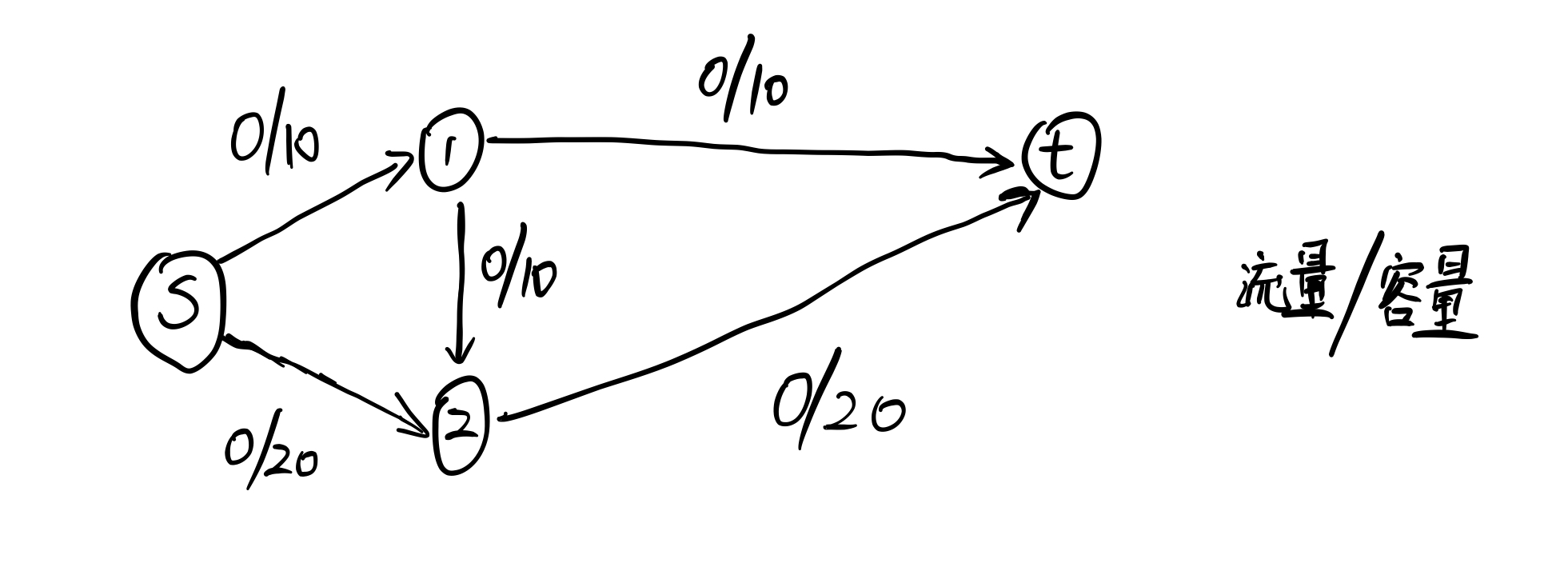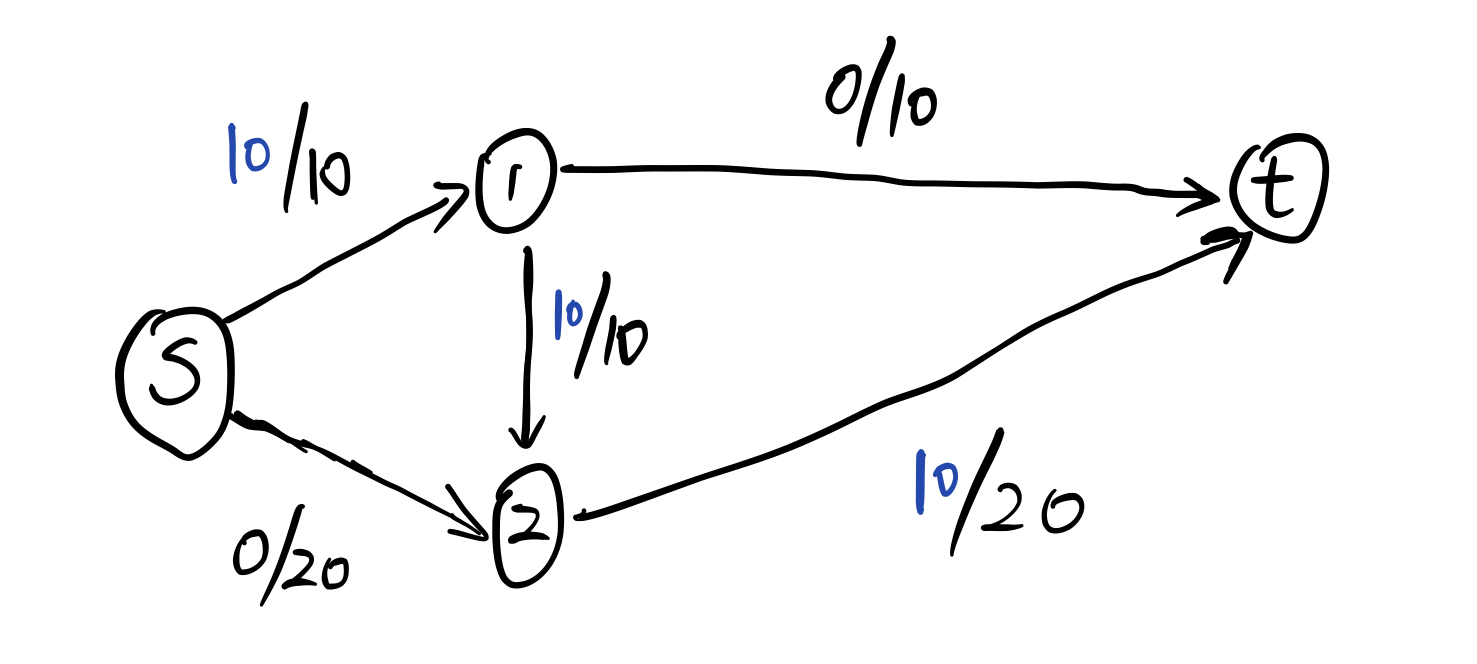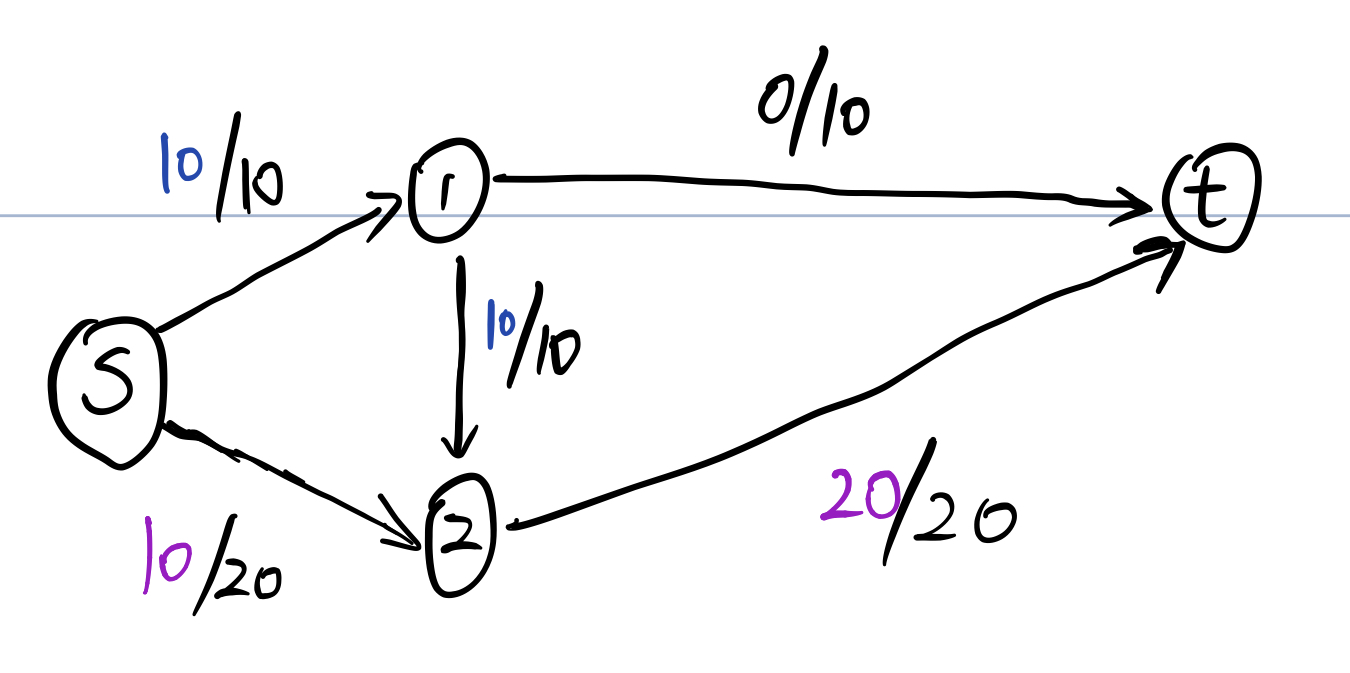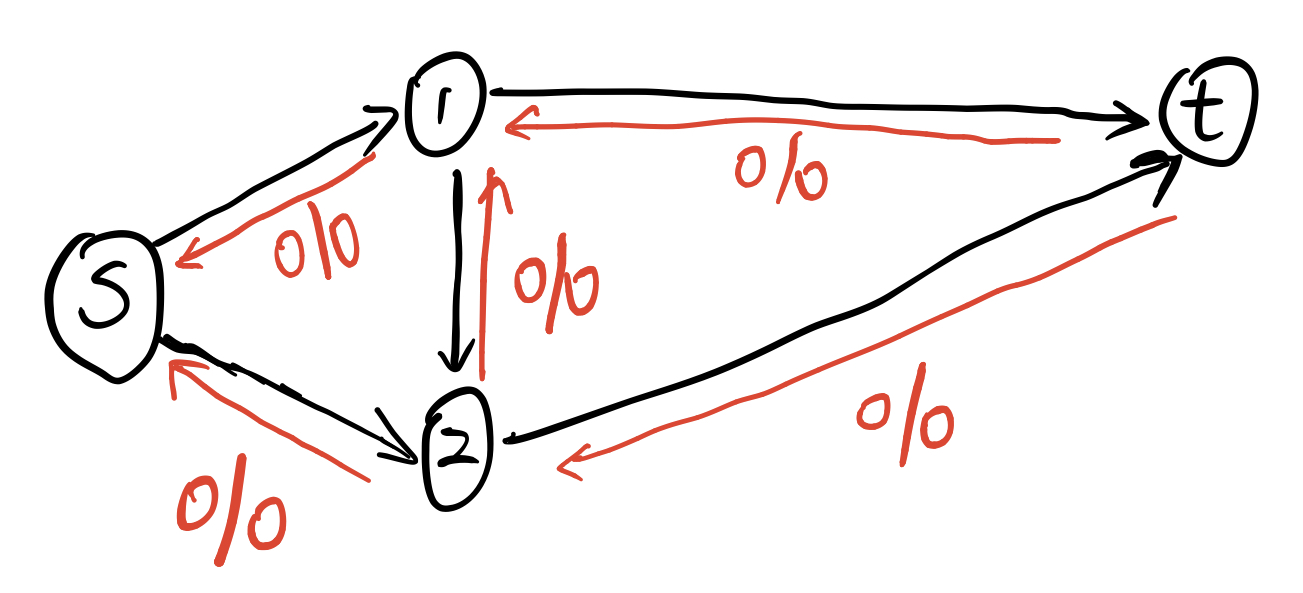# 经典建图

## 无源汇可行流（循环流）

• 对于 $a[i] > 0$，建立 $(ss, i)$ 容量为 $a[i]$ 的边。

$a[i] > 0$ 表示 $i$ 点流入的流量多于流出的流量，那我们需要在残余网络上表示出来这个多余的流量，因此建立 $(ss,i)$ 的边，表示 $i$ 点会多进入一些流量。如果最后这个边可以跑满，说明多余的流量可以找到出路，从而可以达到流量平衡。

• 对于 $a[i] < 0$， 建立 $(i,tt)$ 容量为 $-a[i]$ 的边

• 对于原图中的边 $(u, v, low, high)$，建立 $(u,v)$ 容量为 $high - low$ 的边。

• 跑 $ss$ 到 $tt$ 的最大流，如果从 $ss$ 出去的边（进入 $tt$ 的边）全部满流，则证明有解，否则无解。

## 有源汇可行流

• 设源点为 $s$，汇点为 $t$。

• 建立一条 $(t,s)$ 容量为无穷大的边。

• 再按照无源汇可行流的方式建图。

• 跑 $ss$ 到 $tt$ 的最大流，如果从 $ss$ 出去的边（进入 $tt$ 的边）全部满流，则证明有解，否则无解。

## 有源汇上下界最大流

• 按照有源汇可行流的方式建图。

• 跑 $ss$ 到 $tt$ 的最大流，如果从 $ss$ 出去的边（进入 $tt$ 的边）全部满流，则证明有解，否则无解。

• 再跑 $s$ 到 $t$ 的最大流，得到的流量即为答案。

• 会不会有的边退流之后就不满足最后的流量平衡了？

• 是否需要去掉源点汇点和 $(t,s)$ 这条边？

## 有源汇上下界最小流

• 按照无源汇可行流进行建图
• 跑 $ss$ 到 $tt$ 的最大流
• 建立 $(t,s)$
• 再跑 $ss$ 到 $tt$ 的最大流，$(t,s)$ 这条边上的流量就是答案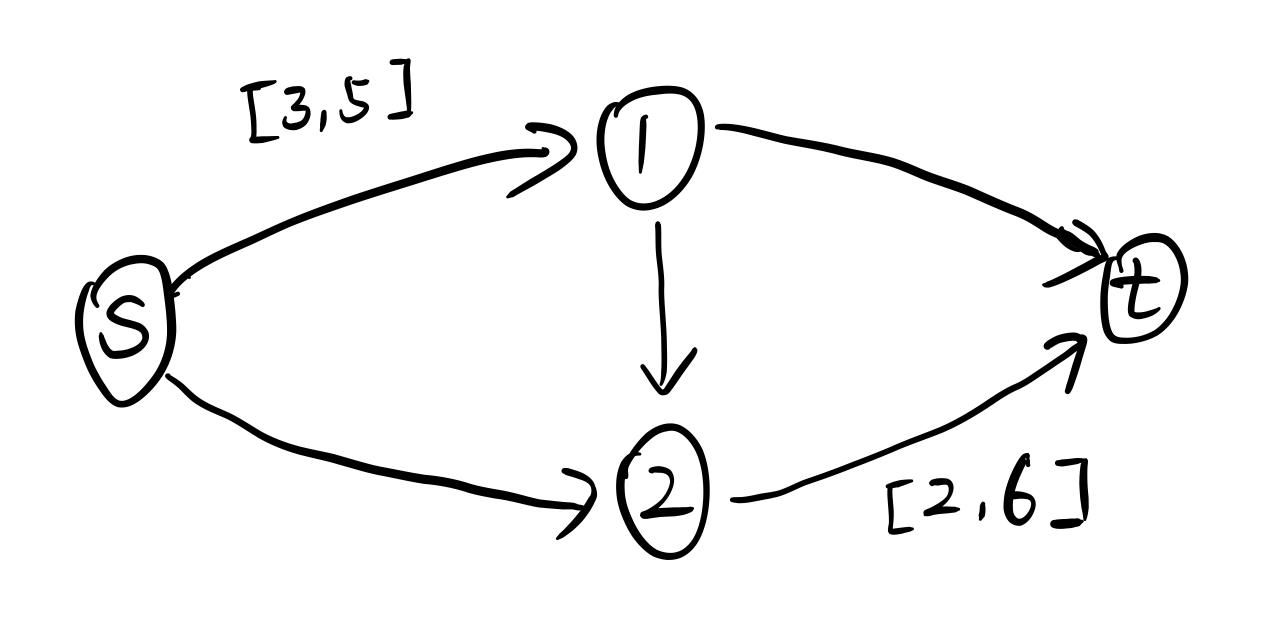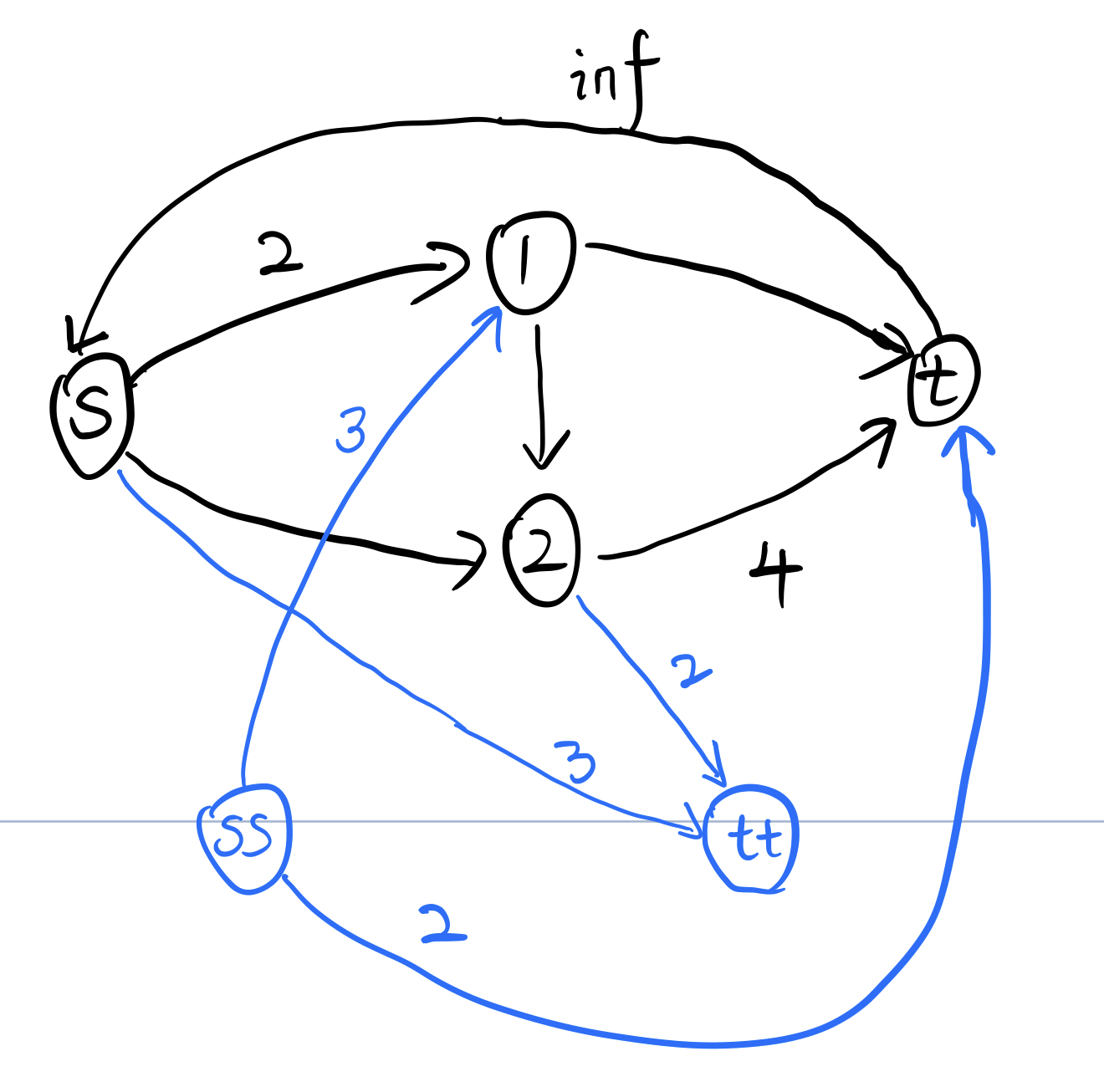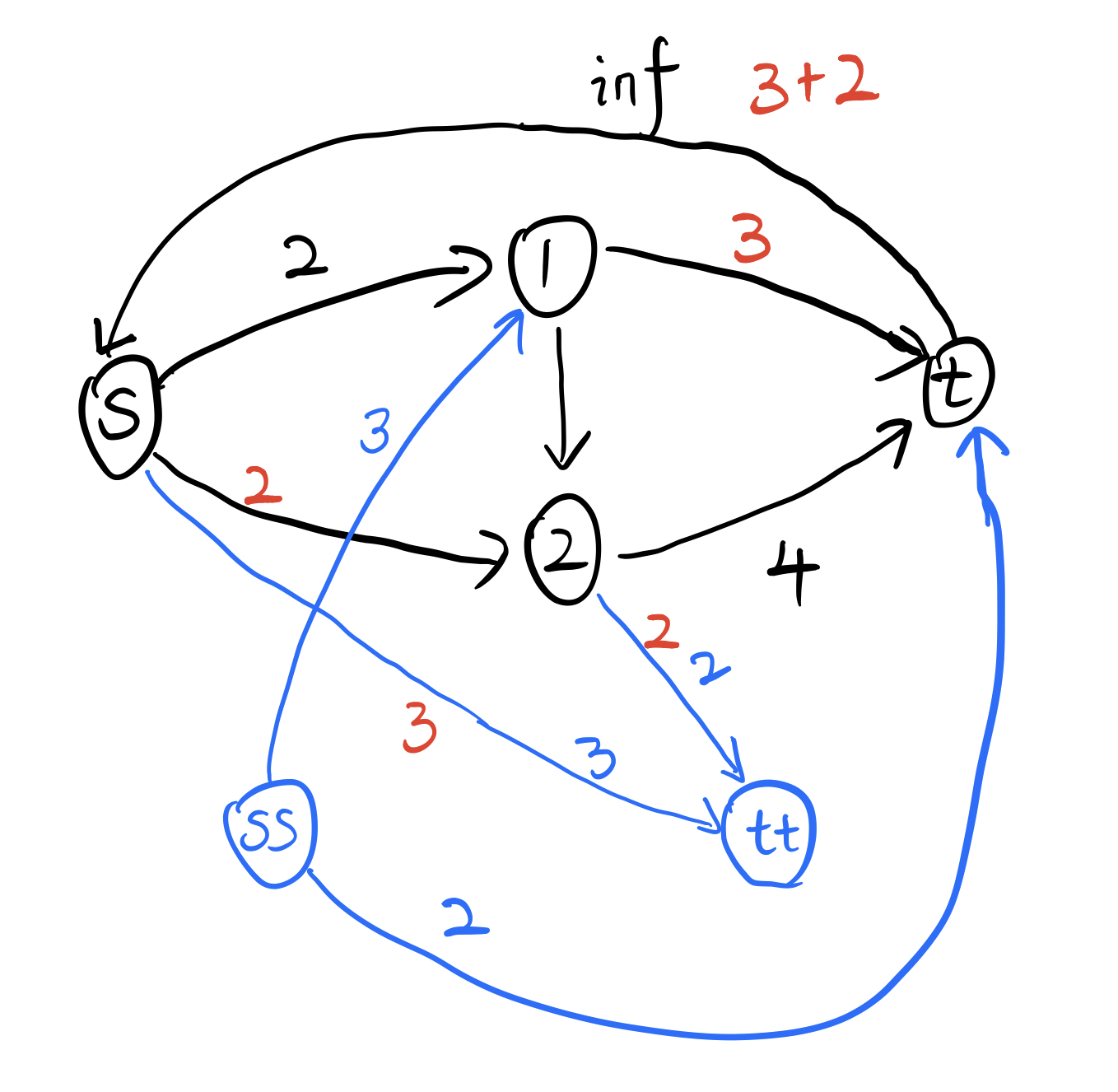$1$ 号点本可以直接走到 $2$ 点然后到达 $tt$ 点，但是它却经过了 $(t,s)$ 这条边绕了一圈才到 $tt$ 点。然而 $(t,s)$ 这条边的流量表示的是最后可行流的大小，所以想要获得最小流，那么应该让通过 $(t,s)$ 边的流量尽可能地少。

## 最大权闭合子图

• $s$ 向所有正权点连边，容量为权值。
• 所有负权点向 $t$ 连边，容量为权值的绝对值。
• 其他的边正常连，容量为正无穷。
• 跑 $s$ 到 $t$ 的最大流，答案为所有正权点的权值和减去最大流。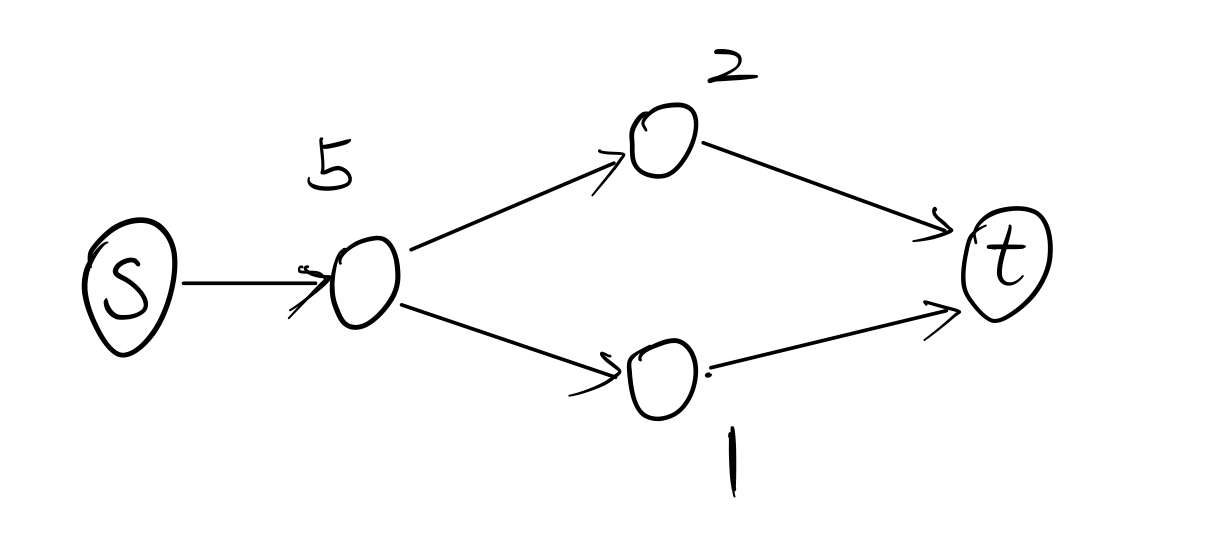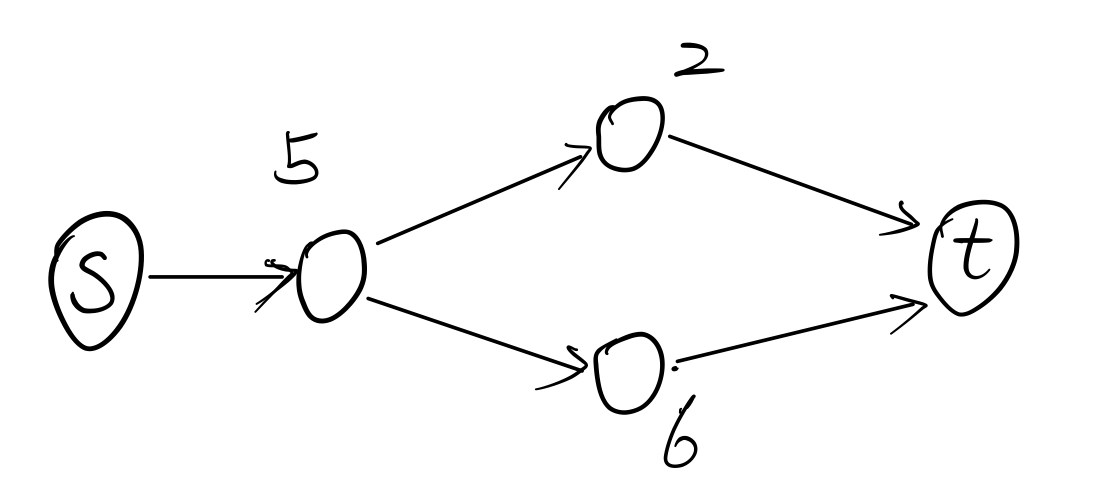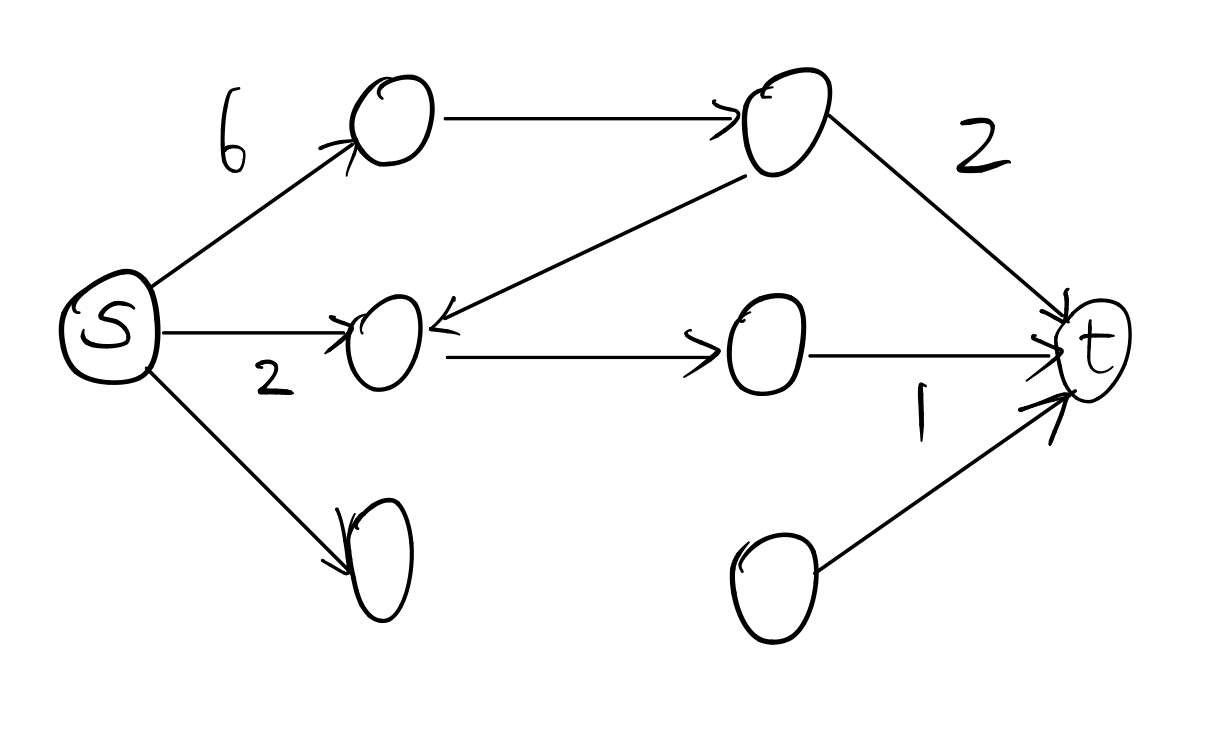## 最大密度子图

$$D = \frac{|E|}{|V|}$$

• 源点向每条边建立容量为 $1$ 的边。
• 每条边向其两个顶点建立无穷大的边。
• 每个顶点向汇点建立容量为 $g$ 的边。
• 跑 $s$ 到 $t$ 的最大流，答案为 $|E| - flow$。

## 拆点与拆边

### 拆边

#### 例题

$n$ 个城市，$m$ 条有向路，现在要运送 $k$ 个货物从 $1$ 号城市到 $n$ 号城市。每条路有个系数 $a_i$，和一个流量上限 $c_i$，运送 $x$ 个货物的花费为 $a_ix^2$。现在求运送完成的最小花费。

$c_i \leq 5$